# Algebra II: Exponential and Logarithmic Functions Chapter Exam

Exam Instructions:

Choose your answers to the questions and click 'Next' to see the next set of questions. You can skip questions if you would like and come back to them later with the yellow "Go To First Skipped Question" button. When you have completed the practice exam, a green submit button will appear. Click it to see your results. Good luck!

Answered 0 of 30

### Page 2

#### Question 10 10. Use the following information to answer the question.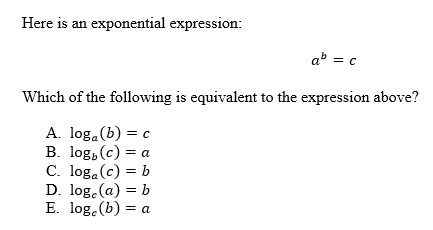### Page 3

#### Question 12 12. Evaluate the logarithm below to 2 decimal places.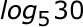#### Question 13 13. Evaluate.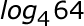#### Question 15 15. Evaluate.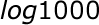### Page 4

#### Question 17 17. Solve, to 2 decimal places, the exponential equation below for x: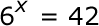#### Question 18 18. Expand the logarithm: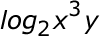#### Question 19 19. Solve the exponential equation below for x: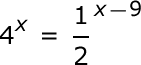### Page 5

#### Question 21 21. Evaluate the logarithm below: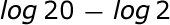#### Question 22 22. Solve the exponential equation below for x: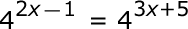#### Question 23 23. Evaluate the logarithm below: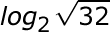#### Question 24 24. Which logarithmic property is shown below?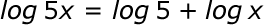### Page 6

#### Question 26 26. Solve the exponential equation below for x: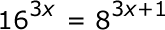#### Question 28 28. Evaluate.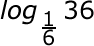#### Question 30 30. Condense the logarithm below: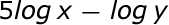#### Algebra II: Exponential and Logarithmic Functions Chapter Exam Instructions

Choose your answers to the questions and click 'Next' to see the next set of questions. You can skip questions if you would like and come back to them later with the yellow "Go To First Skipped Question" button. When you have completed the practice exam, a green submit button will appear. Click it to see your results. Good luck!

Support